# Data Science Questions and Answers (Questions 11 to 20)

You can find Question 1 to 10 here.

So let’s get started!

##### 11. What is Multivariate Linear Regression?

This is a type of regression were the target variable(or dependent variable) Y is a linear function of multiple predictor (independent) variables.

For example, given the variables x1, x2, … , xk

We seek to model the relationship: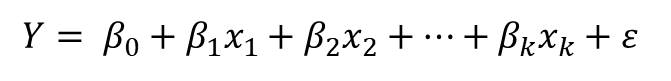##### Stepwise Selection

The Forward Selection method successively selects a list of predictor variables that have the highest correlation coefficient with the response variable. So it starts with a model containing no predictor variables. Then it adds the predictor variable one at a time until all predictor variables are added. For each step, we calculate the F-statistic to determine if the strongest linear relationship have been obtained. If yes, the procedure stops, if no, continue with the next predictor variable.

The Backward Selection (or backward elimination) works exactly in reverse of the forward selection. Here, we start with the full least-squares model that contains all the predictor variables. Then it iteratively eliminates the least-useful predictor variable one at a time.

In both the forward selection and backward elimination, the strength of the relationship between the predictor and response variable is determined by calculating the F-statistic.

The Stepwise Selection method is like a combination of both methods discussed above. It both adds and removes variables from the model in steps. After adding a variable, the method may also remove a variable if the variable is not longer contributes to the model fit.

##### 13. Briefly explain the term multicolinearity

Multicolinearity is an occurrence in multivariate linear regression  where a linear relationship exists between two or more of the independent variables. As such, the estimation of the regression coefficients becomes  unreliable and sometimes it becomes hard to perform an analysis.

The effect  of multicolinearity is determined using variation inflation factor (VIF) which is given by: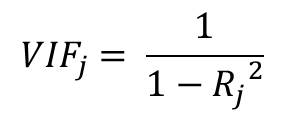where Rj is the coefficient of multiple correlation on the jth variable

##### 14. What is heteroscedasticity?

Heteroscedasticity refers to a systematic change in the spread of the residual (or error term) over  a range of measured values. Another definition: it is the linear correlation between two or more predictor variables.

This is a challenge because he assumption in regression(using ordinary least squares OLS) is that all the residuals are taken from a population with a constant variance. Therefore the residuals are also expected have constant variance Var(εi) = σ2.

This is called homoscedasticity.

But since this assumption fails in the case of heteroscedasticity, there is unequal scatter as shown below.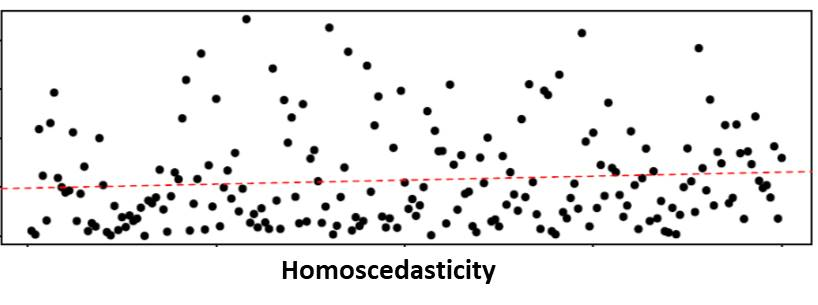One way to  handle this problem is to transform the response function Y using  a concave function such as log Y or root Y.

##### 15. Write briefly on Sensitivity Analysis

I would just give you the simple and clear definition.

Sensitivity Analysis is the study of the relationship between the uncertainty in the output of a model and the uncertainty in the inputs.

##### 16. Differentiate between Factor Analysis (FA) and Principal Components Analysis (PCA)

Principal Components Analysis (PCA) is concerned with explaining the variance between variables while Factor Analysis (FA) in concerned with explaining the covariance among variables.

So PCA uses the total variance  while Factor analysis uses the shared or common variance between factors.

Again while FA is interested in identifying latent variables, PCA tries to perform a linear combination of existing variable.

##### 17. What is Measure of Sampling Adequacy (MSA)?

Before Factor Analysis can be performed on a dataset, there must be some correlation between the predictor variables in the data. So if there is strong correlation between the variables included in the study, then the data would likely be suitable for factor analysis.

MSA is a measure of how suitable a data set is for Factor Analysis and is defined using he KMO index explained in Question 18.

Other ways of checking sampling adequacy includes:

• Visual Inspection for r> .3
• Partial correlation (correlation between variables when the effect of other variables are taken into account and partialed out). Its expected to be small
• anti-image correlation: negative value of the partial correlation.
• Bartlett test of sphericity: provides statistical probability that correlated matrix has significant correlation among some of the variables

##### 18. What is the KMO Statistic?

KMO or Kaiser-Meyer-Olkin statistic is a value that measures sampling adequacy. It takes a value from 0 to 1. Values close to 0 is considered not suitable, while values close to 1 is acceptable.

In summary, KMO provides the following:

• 0     – 0.49 unacceptable.
• 0.5 – 0.59 miserable.
• 0.6 – 0.69 mediocre.
• 0.7 – 0.79 middling.
• 0.8 – 0.89 meritorious.
• 0.9 – 1.00 marvelous.

##### 19. Differentiate between Communality and Uniqueness

Communality and Uniqueness are terms in Factor analysis used to describe variances.

Communality is the portion of the variance that is contributed by common factors

Uniqueness is the part of the variance that is not explained by the common factors.

So given that the total variance is: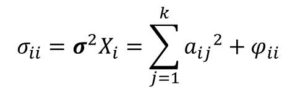The the term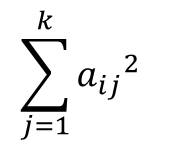is the communality

while the term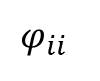is the uniqeness

##### 20. What are the steps of Principal Components Analysis?

Principal Components Analysis takes the following steps:

• Compute the covariance matrix
• Compute the eigen vectors
• Choose a dimension k (number of components)
• Define the dimension of the reduced data set#### kindsonthegenius

Kindson Munonye is currently completing his doctoral program in Software Engineering in Budapest University of Technology and Economics

View all posts by kindsonthegenius →